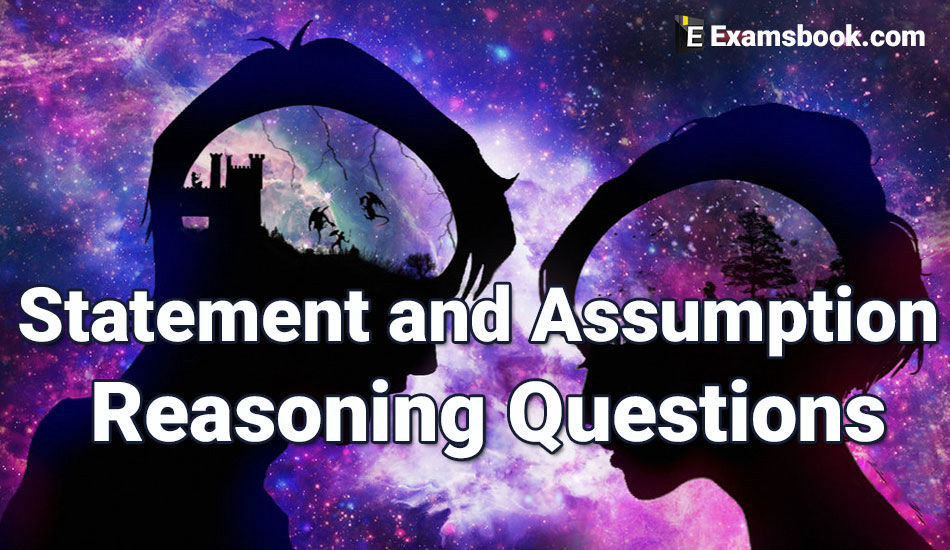• Save

# Statement and Assumption Reasoning Questions and Answers

Vikram SinghLast year 15.6K ViewsStatement and Assumption questions are mostly asked in competitive exams. So students should practice Statement and Assumption questions and answers who doing preparation for SSC, Bank and other competitive exams.

Here are the very crucial and useful questions and answers of Statement and Assumption for competitive exams. Do practice of these selective statements and Assumption questions to get good marks in your exams.

Here, students can practice with the various statement and assumption in Hindi questions who want to practice in hindi.

Candidates should solve useful Statement and Argument questions here for exams. You can read also Statement and Conclusion questions with answers.

## Important Statement and Assumption questions:

Direction:(1-3)

As per the given statement

1. Statement: Most of the college students are found more interested in fashion than the study.

Actions:

I. The colleges should ban the use of fashionable clothes & other products.

II. The college students should be busy in study so that they will not have the extra time for fashion & other things.

(A) Only assumption I is correct.

(B) Only assumption II is correct.

(C) Assumption I & II – both are correct.

(D) None of assumption I & II is correct.

Ans .   C

2. Statement: Recently the counting of a leopard was done & found that some of the leopards are missing from the leopard reserve.

Actions

I. The government should arrange strictly for saving the remaining leopards.

II. The government should immediately find the criminals responsible for the missing of leopards.

(A) Only assumption I is correct.

(B) Only assumption II is correct.

(C) Assumption I & II – both are correct.

(D) None of assumption I & II is correct.

Ans .   C

3. Statement: No. of malaria cases in the city is increased since last few months.

Actions:

I. The municipal officials should take an immediate action to destroy the mosquito breeding places & improve the cleanliness.

II. The mission should be started to train the people with the help of local media & social workers for the measures of prevention of the disease.

(A) Only assumption I is correct.

(B) Only assumption II is correct.

(C) Assumption I & II – both are correct.

(D) None of assumption I & II is correct.

Ans .   C

In the following question, a statement is given & then two assumptions – I & II are given. You have to consider the statement as true though it seems to be different from the actual facts, then determine which of assumption/s can be definitely correct. Select the option your answer.

4. The victim of imbalance in the industrial development is generally illiterate & economically weaker people.

Assumptions:

I. The industrial development in India is mainly according to the political thinking.

II. The scheduled tribe people are always adversely affected by the industrial development.

(A) Only assumption I is correct.

(B) Only assumption II is correct.

(C) Assumption I & II – both are correct.

(D) None of assumption I & II is correct.

Ans .   D

Two assumptions are given after a statement. You have to determine which of the assumption is correct. Mention your answer.

5. Statement: Rich people are very commonly suffering from heart attack.

Assumptions:

I. Most of the deaths of rich people are due to heart attack.

II. Poor people do not suffer the heart attack.

(A) Only assumption I is correct.

(B) Only assumption II is correct.

(C) Assumption I & II – both are correct.

(D) None of assumption I & II is correct.

Ans .   A

6. Consider the statement true: There are books in each library.” Which of the following statements is definitely true?

(A) Books are only in the libraries.

(B) None of a library is without books.

(C) There are no readers in some libraries.

(D) Libraries are for books only.

Ans .   B

7. Statement: Our digest system gets affects because of the difficulties & fast life.

Assumptions:

I. Life is full of difficulties.

II. We do not have the security system of ancient time.

(A) Only assumption I is correct.

(B) Only assumption II is correct.

(C) Assumption I & II – both are correct.

(D) None of assumption I & II is correct.

Ans .   A

8. Statement: Quality of life is lowered in India because of the population bombardment.

Assumptions:

I. Population & quality of life are related to each other.

II. Quality of life in India is not related to the population.

(A) Only II is correct.

(B) I & II – both are correct.

(C) Only I is correct.

(D) I & II – both are not correct.

Ans .   C

Ask me in the comment section, if you face any problem while solving Statement and Assumption questions. Visit next page for more Statement and Assumption problems.

### Vikram Singh

Providing knowledgable questions of Reasoning and Aptitude for the competitive exams.

## Report Error: Statement and Assumption Reasoning Questions and Answers